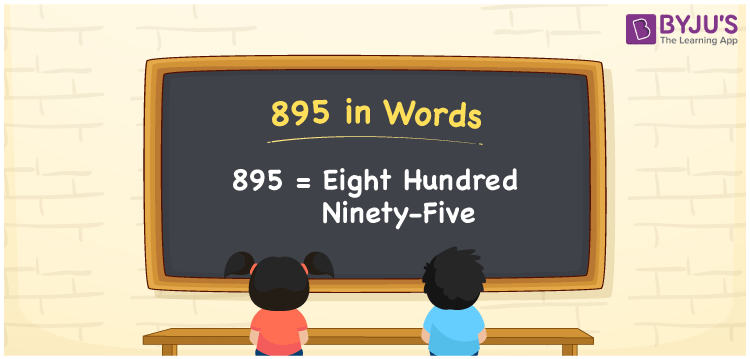# 895 in words

895 in words is written as Eight Hundred and Ninety Five. 895 represents the count or value. The article on Counting Numbers can give you an idea about count or counting. The number 895 is a 3 digit number that is used in expressions related to money, days, distance, length, weight and so on. Let us consider an example for 895. “This school bag costs Eight Hundred and Ninety Five rupees.”

 895 in words Eight Hundred and Ninety Five Eight Hundred and Ninety Five in Numbers 895

## 895 in English Words## How to Write 895 in Words?

We can convert 895 to words using a place value chart. The number 895 has 3 digits, so let’s make a chart that shows the place value up to 3 digits.

 Hundreds Tens Ones 8 9 5

Thus, we can write the expanded form as:

8 × Hundred + 9 × Ten + 5 × One

= 8 × 100 + 9 × 10 + 5 × 1

= 895

= Eight Hundred and Ninety Five.

895 is the natural number that is succeeded by 894 and preceded by 896.

895 in words – Eight Hundred and Ninety Five.

Is 895 an odd number? – Yes.

Is 895 an even number? – No.

Is 895 a perfect square number? – No.

Is 895 a perfect cube number? – No.

Is 895 a prime number? – No.

Is 895 a composite number? – Yes.

## Solved Example

1. Write the number 895 in expanded form

Solution: 8 × 100 + 9 × 10 + 5 × 1

We can write 895 = 800 + 90 + 5

= 8 × 100 + 9 × 10 + 5 × 1.

## Frequently Asked Questions on 895 in words

Q1

### How to write the number 895 in words?

895 in words is written as Eight Hundred and Ninety Five.
Q2

### Is 895 divisible by 5?

Yes. 895 is divisible by 5.
Q3

### Is 895 a prime number?

No. 895 is not a prime number.